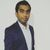# QlikView App Dev

Discussion Board for collaboration related to QlikView App Development.

Announcements
On May 18th at 10AM EDT we will answer your QlikView questions live. REGISTER
cancel
Showing results for
Did you mean:Master

## Logic required

Hi guys

I need last column

 A B C Result Cat 10 1 10 Tiger 54 2 10 Divide by 2 Dog 56 3 (10 Divide by 2)/3 Rabbit 34 4 ((10 Divide by 2)/3)/4 Pug Boss 43 5 (((10 Divide by 2)/3)/4)/5

Thanks

1 Solution

Accepted SolutionsMVP

May be this

=If(RowNo() = 1, B, Above(Result)/C)

or this

=If(RowNo() = 1, B, Above(Column(1))/C)4 RepliesCreator II

=(Result / Alt(above(Result),1))Master
Author

Not workingMVP

May be this

=If(RowNo() = 1, B, Above(Result)/C)

or this

=If(RowNo() = 1, B, Above(Column(1))/C)Master
Author

Thanks Sunny, got my mistake. small bracket played all the game